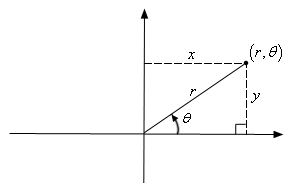JavaScript 数学曲线—连锁螺线

简介• r ：径向距离。

• a ：常数。

• θ ：极角。

绘制x = rcos(θ)

y = rsin(θ)

θ = arctan(y/x)function draw() {

let a = 100, angle = 0.1;

let x = 0, y = 0, points = [];

const acceleration = 0.1, circleNum = 20;

while (angle <= circleNum * 2 * Math.PI) {

const angleSqrt = Math.sqrt(angle);

x = (a / angleSqrt) * Math.cos(angle);

y = (a / angleSqrt) * Math.sin(angle);

points.push([x, y]);

angle = angle + acceleration;

}

// 实现把点绘制成线的方法

line({ points: points});

}

参考资料